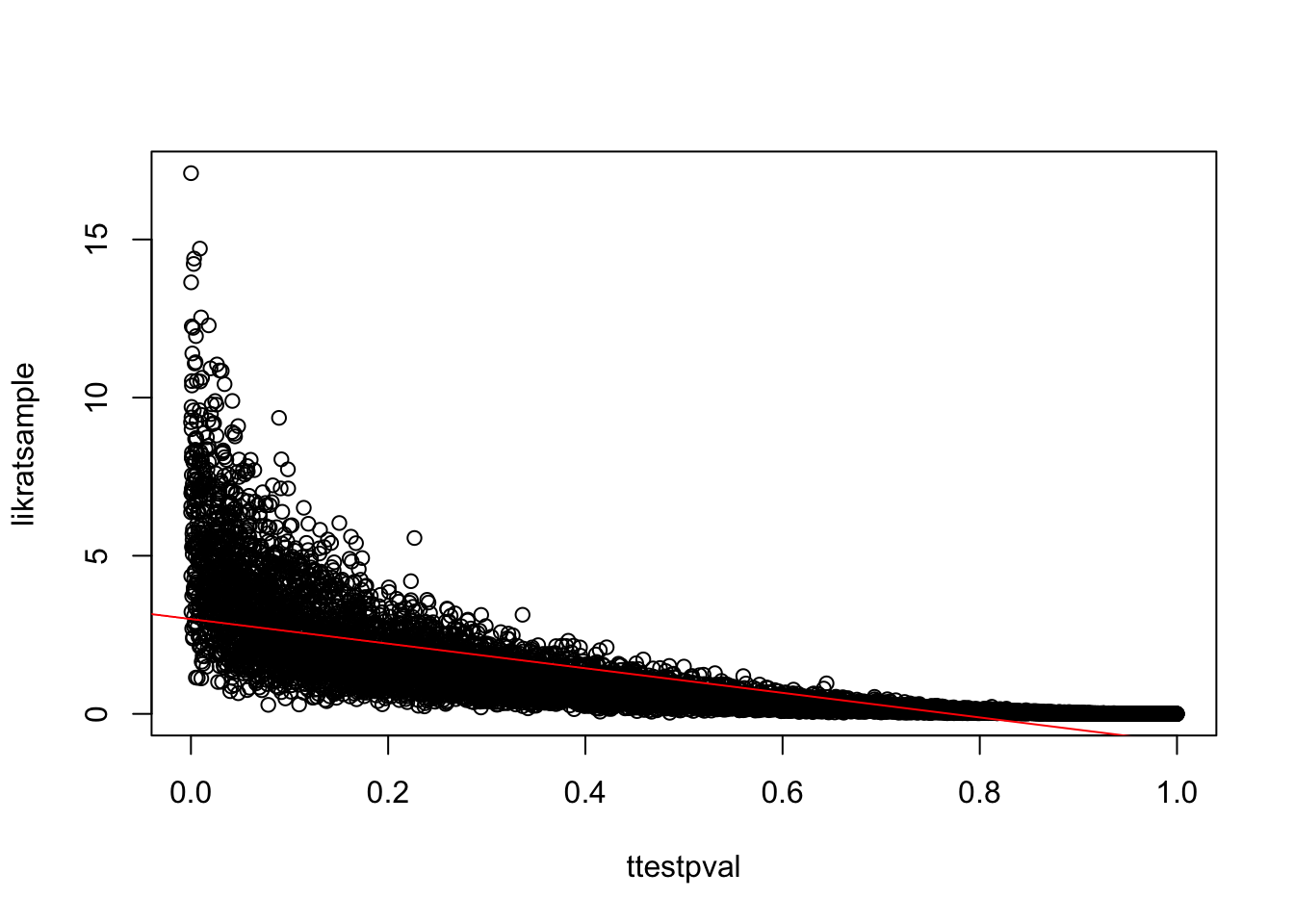[Thanks to Scott Glover for comments]

Someone said to me: The smaller the p-value, the higher the likelihood under the alternative.’’

They probably mean:

The smaller the p-value, the higher the likelihood ratio under the alternative vs the null.’’

This statement ignores the fact that under low power conditions, 100% of the significant effects will be based on overestimates of the true effect. This is what Gelman’s Type M error is all about, and it is a shocking fact, which I demonstrate below.

Suppose the alternative is that $$\mu = 0.1$$, let sd = 1. Thus, we assume here that this specific alternative is in fact true.

Power is low for sample size 10:

mu<-0.1
power.t.test(d=mu,n=10,sd=1,alternative="two.sided",type="one.sample",strict=TRUE)
##
##      One-sample t test power calculation
##
##               n = 10
##           delta = 0.1
##              sd = 1
##       sig.level = 0.05
##           power = 0.0592903
##     alternative = two.sided

Simulate some data and compute likelihood ratio Ha/H0 using the true value of 0.1, and using the sample mean. The latter is what we normally do.

nsim<-10000
ttestpval<-likratsample<-means<-rep(NA,nsim)
for(i in 1:nsim){
y <- rnorm(10,mean=mu,sd=1)
ttestpval[i]<-t.test(y)$p.value means[i]<-mean(y) ## likelihood ratio under sample mean: likratsample[i]<- -2*log(prod(dnorm(y,mean=0,sd=1))/prod(dnorm(y,mean=means[i],sd=1))) } Create a data frame with sample means and p-values, and likelihoods: (criticalval<-qchisq(0.05,df=1,lower.tail=FALSE)) ##  3.841459 likratpval<-pchisq(likratsample,df=1,lower.tail=FALSE) dat<-data.frame(means,ttestpval,likratsample,likratpval) head(dat) ## means ttestpval likratsample likratpval ## 1 0.05127913 0.9030325 0.02629549 0.8711808 ## 2 0.46804303 0.1630380 2.19064275 0.1388514 ## 3 0.17049681 0.6508300 0.29069161 0.5897777 ## 4 0.24739473 0.4041785 0.61204151 0.4340202 ## 5 0.11691442 0.7022102 0.13668981 0.7115942 ## 6 0.09655920 0.7593891 0.09323679 0.7601019 Earlier I wrote incorrectly: Note that the t-test based p-values will be significant more often compared to the likelihood based p-values.’’ Actually, the t-test and likelihood ratio test are completely identical (see the Casella and Berger textbook). So they should deliver the same proportion of significant results: mean(dat$ttestpval<0.05)
##  0.0612
mean(dat$likratpval<0.05) ##  0.0662 What we also see is that the t-test p-value is strongly correlated with the likelihood ratio, as claimed. plot(likratsample~ttestpval) abline(lm(likratsample~ttestpval),col="red")If we focus only on likelihoods associated with significant effects, the likelihood ratio is correlated with p-values computed using the t-test: dat<-subset(dat,ttestpval<0.05) plot(dat$ttestpval,dat$likratsample) abline(lm(dat$likratsample~dat\$ttestpval))